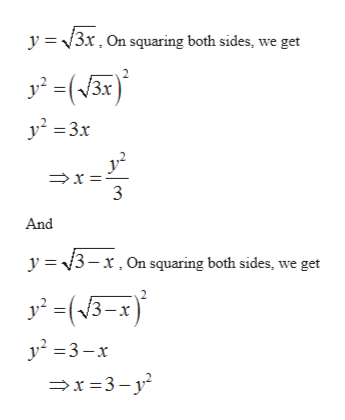# Find the volume when the region bounded by the y=√(3x) and y=√(3−x) is rotated about the x axis.

Question
22 views

Find the volume when the region bounded by the y=√(3x) and y=√(3−x) is rotated about the x axis.

check_circle

Step 1

To calculate the volume of the region bounded by the given curves when rotated around x-axis.

Step 2

To find the volume of revolution, we will use the Shell method. The region bounded by the curves when rotated about x-axis, it gives the solid shown as below:

Step 3

Since the region bounded between the curves is easy to integrate with respect to the vari...help_outlineImage Transcriptionclosey 3r. On squaring both sides, we get y23x And y 3-x.On squaring both sides, we get -x y2 3-x x3-y fullscreen

### Want to see the full answer?

See Solution

#### Want to see this answer and more?

Solutions are written by subject experts who are available 24/7. Questions are typically answered within 1 hour.*

See Solution
*Response times may vary by subject and question.
Tagged in

### Integration